# Finding the Area of Rectangular Figures in the Real World: 3rd Grade Math Lesson

Lesson Objective: The lesson is aligned to the Common Core State Standards for Mathematics – 3.MD.7b Geometric Measurement – Multiply side lengths to find areas of rectangles in context of solving real world and mathematical problems.

Materials Required: graph paper, calculator

## Lesson Procedure: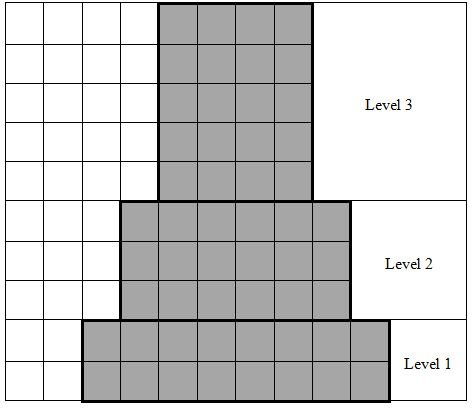Finding Area of Rectangular Figures in Real World Mathematical Problems

The drawing of the front view of a tower is shown on the graph paper below. The tower has 3 levels. Each level is the shape of a rectangular figure. Each unit is equal to one meter.

1. Identify the lengths of the two sides of the rectangular figures.

2. Find the areas of the rectangular figures.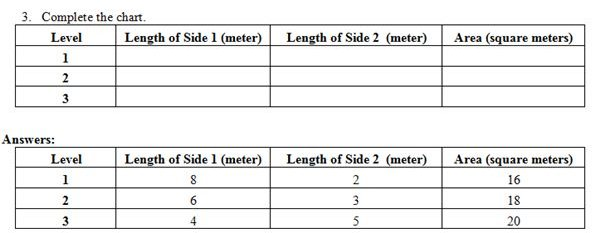3. Complete the chart.

## Individual or Group Work: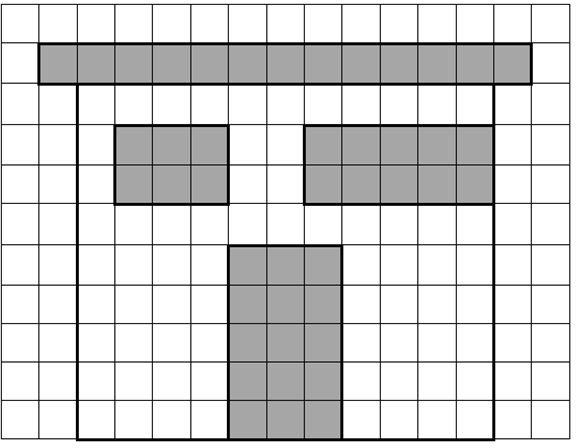The drawing of the front view of a house is shown on the graph paper at right. The house has a door, 2 windows, and a roof. All the shapes are rectangular figures. Each unit is equal to one meter.

1. Identify the lengths of the two sides of the rectangular figures.

2. Find the areas of the rectangular figures.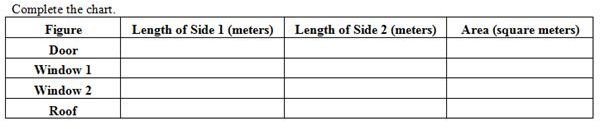Complete the chart

3. Rhys’ bedroom is in the shape of a rectangle. The length of the bedroom is 10 feet. The width of the bedroom is 12 feet. What is the area of the bedroom, in square feet?

4. Jasper has a pond in his back yard. The pond is in the shape of a rectangle. The length of the pond is 6 feet. The width of the pond is 8 feet. What is the area of the pond, in square feet?

5. Jack planted a flower garden. The garden is in the shape of a rectangle. The length of the garden measures 4 feet. The width of the garden measures 5 feet. What is the area of the garden, in square feet?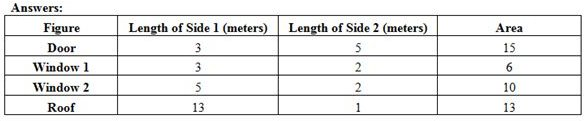The area of Rhys’ bedroom is 120 square feet.
The area of Jasper’s pond is 48 square feet.
The area of Jack’s garden is 20 square feet.

Students should be able to determine the area of rectangular figures in real world and mathematical problems by multiplying the two sides of the rectangular figures or the lengths and the widths of rectangular figures.